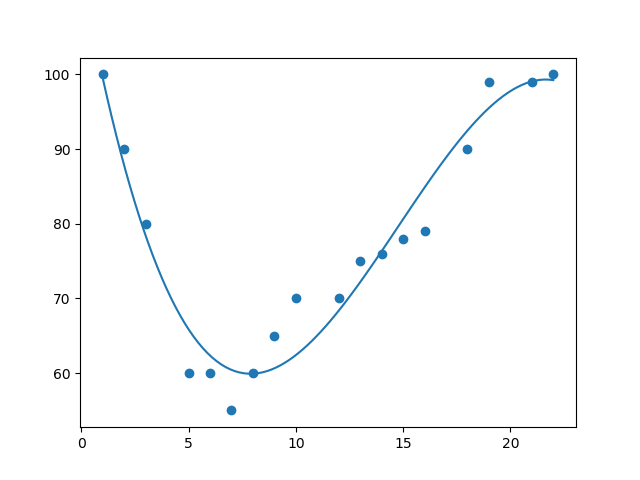# Linear Regressions

A Regression is a method to determine the relationship between one variable (y) and other variables (x).

In statistics, a Linear Regression is an approach to modeling a linear relationship between y and x.

In Machine Learning, a Linear Regression is a supervised machine learning algorithm.

## Scatter Plot

This is the scatter plot (from the previous chapter):

### Example

const xArray = [50,60,70,80,90,100,110,120,130,140,150];
const yArray = [7,8,8,9,9,9,10,11,14,14,15];

// Define Data
const data = [{
x:xArray,
y:yArray,
mode: "markers"
}];

// Define Layout
const layout = {
xaxis: {range: [40, 160], title: "Square Meters"},
yaxis: {range: [5, 16], title: "Price in Millions"},
title: "House Prices vs. Size"
};

Plotly.newPlot("myPlot", data, layout);
Try it Yourself »

## Predicting Values

From the scattered data above, how can we predict future prices?

• Use hand drawn linear graph
• Model a linear relationship
• Model a linear regression

## Linear Graphs

This is a linear graph predicting prices based on the lowest and the highest price:

### Example

const xArray = [50,60,70,80,90,100,110,120,130,140,150];
const yArray = [7,8,8,9,9,9,9,10,11,14,14,15];

const data = [
{x:xArray, y:yArray, mode:"markers"},
{x:[50,150], y:[7,15], mode:"line"}
];

const layout = {
xaxis: {range: [40, 160], title: "Square Meters"},
yaxis: {range: [5, 16], title: "Price in Millions"},
title: "House Prices vs. Size"
};

Plotly.newPlot("myPlot", data, layout);
Try it Yourself »

## From a Previous Chapter

A linear graph can be written as y = ax + b

Where:

• y is the price we want to predict
• a is the slope of the line
• x are the input values
• b is the intercept

## Linear Relationships

This Model predicts prices using a linear relationship between price and size:

### Example

const xArray = [50,60,70,80,90,100,110,120,130,140,150];
const yArray = [7,8,8,9,9,9,10,11,14,14,15];

// Calculate Slope
let xSum = xArray.reduce(function(a, b){return a + b;}, 0);
let ySum = yArray.reduce(function(a, b){return a + b;}, 0);
let slope = ySum / xSum;

// Generate values
const xValues = [];
const yValues = [];
for (let x = 50; x <= 150; x += 1) {
xValues.push(x);
yValues.push(x * slope);
}
Try it Yourself »

In the example above, the slope is a calculated average and the intercept = 0.

## Using a Linear Regression Function

This Model predicts prices using a linear regression function:

### Example

const xArray = [50,60,70,80,90,100,110,120,130,140,150];
const yArray = [7,8,8,9,9,9,10,11,14,14,15];

// Calculate Sums
let xSum=0, ySum=0 , xxSum=0, xySum=0;
let count = xArray.length;
for (let i = 0, len = count; i < count; i++) {
xSum += xArray[i];
ySum += yArray[i];
xxSum += xArray[i] * xArray[i];
xySum += xArray[i] * yArray[i];
}

// Calculate slope and intercept
let slope = (count * xySum - xSum * ySum) / (count * xxSum - xSum * xSum);
let intercept = (ySum / count) - (slope * xSum) / count;

// Generate values
const xValues = [];
const yValues = [];
for (let x = 50; x <= 150; x += 1) {
xValues.push(x);
yValues.push(x * slope + intercept);
}
Try it Yourself »

## Polynomial Regression

If scattered data points do not fit a linear regression (a straight line through the points), the data may fit an polynomial regression.

A Polynomial Regression, like linear regression, uses the relationship between the variables x and y to find the best way to draw a line through the data points.W3Schools is optimized for learning and training. Examples might be simplified to improve reading and learning. Tutorials, references, and examples are constantly reviewed to avoid errors, but we cannot warrant full correctness of all content. While using W3Schools, you agree to have read and accepted our terms of use, cookie and privacy policy.